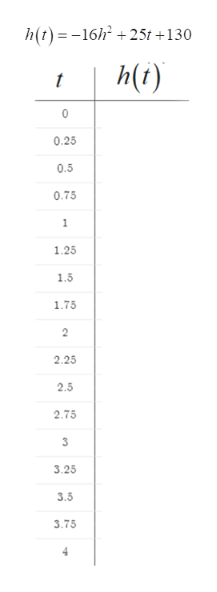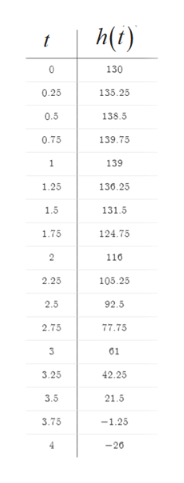# If we stand on top of a 130-foot building and throw a ball up into the air with an initial velocity of 25 feet per second, then the height of the ball above the ground is given by the following.H = −16t2 + 25t + 130Here t is time in seconds, and H is measured in feet.(a) Complete the following table giving the value H at time t.tH0      0.25 0.5   0.75 1      1.25 1.5   1.75 2      2.25 2.5   2.75 3      3.25 3.5   3.75 4      (c) Find the value of t that maximizes H. (Round your answer to two decimal places.)t =  sec(d) Find the maximum value of H. (Round your answer to two decimal places.)H =  ft

Question
117 views
If we stand on top of a 130-foot building and throw a ball up into the air with an initial velocity of 25 feet per second, then the height of the ball above the ground is given by the following.
H = −16t2 + 25t + 130
Here t is time in seconds, and H is measured in feet.
(a) Complete the following table giving the value H at time t.
t H
0
0.25
0.5
0.75
1
1.25
1.5
1.75
2
2.25
2.5
2.75
3
3.25
3.5
3.75
4

(c) Find the value of t that maximizes H. (Round your answer to two decimal places.)
t =  sec

(d) Find the maximum value of H. (Round your answer to two decimal places.)
H =  ft

check_circle

Step 1

Part a) Complete the given table

Part b) Determine the value of t for which the height is maximum.

Part c) Determine the maximum height.

Step 2

Given:help_outlineImage Transcriptioncloseh(t) = -16h + 25t +130 h(i) 0.25 0.5 0.75 1.25 1.5 1.75 2.25 2.5 2.75 3.25 3.5 3.75 4. fullscreen
Step 3

Compute the value of the function h(t) for different va...help_outlineImage Transcriptioncloseh(t) 130 0.25 135.25 0.5 138.5 0.75 139.75 139 1.25 130.25 1.5 131.5 1.75 124.75 110 105.25 2.25 2.5 92.5 2.75 77.75 61 3.25 42.25 3.5 21.5 3.75 -1.25 -20 fullscreen

### Want to see the full answer?

See Solution

#### Want to see this answer and more?

Solutions are written by subject experts who are available 24/7. Questions are typically answered within 1 hour.*

See Solution
*Response times may vary by subject and question.
Tagged in

### Algebra